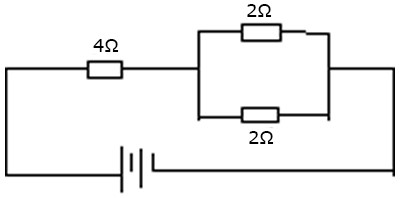Categories

# Arrangement of Resistors and Ohm’s Law

Resistors can be arranged in series and in parallel.

have different potential differences.

When they are connected in parallel, they have the same potential difference but different current.

# Ohm’s Law

The electric current passing through a metallic conductor is directly proportional to the potential difference applied between its end provided temperature and other physical property of the conductor remains constant.

Current =Potential differenceResistanceV=IR

R is a constant of proportionality and depends on the nature of the material. The unit of resistance is ohm.

Calculations

1. A potential difference of 240V is applied to a lamp of 60 ohms resistance. What amount of current will flow in the circuit?

Solution

Current =Potential differenceResistance=24060=4A

1. (a) Calculate the effective resistance in the diagram shown below.(b) Calculate the current flowing in the circuit aboveSolution(a) Solve the parallel first,1RP=1R1+1R21RP=12+121RP=22=1RP=1ΩR=RP+4=1+4=5Ω(b) Total resistance = 5Ω.From Ohm’s law,Current =Potential differenceResistance=205=4A
1. A current of 3A flows in a circuit when a p.d of 24V is applied to it. The resistance across the circuit is
SolutionI=3AV=24VR=?V=IR24=3×RR=243=8Ω